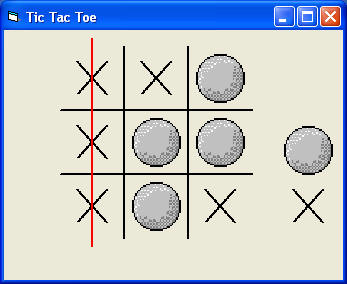# Tic Tac Toe

Tic Tac Toe is a popular game that you can play at any time and anywhere as long as you have a piece of paper and a pen, or you could draw it on sand or any surface. Now, you can create a VB version that allow uers to play the game virtually. First of all, you need to draw the interface with four straight lines, then  insert nine image controls and make them an array, Image1(0) to Image1(8). Secondly,  insert two pictures, one is a circle and the other one is a cross to represent player 1 and player2.

To check whether a position is empty or not, we use two arrays, cross(8) and ball(8) (We use ball instead of circle because circle is an internal function of VB) and set them as Boolean. If cross(n)=false and ball(n)=false, that means position n is not occupied by a cross or a ball, where n is any integer between 0 and 8.

In addition,  you need to insert eight straight lines that would cross out three crosses or three circles if they are aligned in a straight line side by side, as shown in the diagram. You need to make these lines invisible at start-up and make one of the lines appear whenever the above condition is fulfilled.

### The InterfaceDim cross(8) As Boolean
Dim ball(8) As Boolean
Dim m As Integer
Dim player As Integer

Sub check_status()
If ball(0) = True And ball(1) = True And ball(2) = True Then
Line10.Visible = True
End If
If ball(3) = True And ball(4) = True And ball(5) = True Then
Line9.Visible = True
End If
If ball(6) = True And ball(7) = True And ball(8) = True Then
Line8.Visible = True
End If
If ball(0) = True And ball(3) = True And ball(6) = True Then
Line5.Visible = True
End If

If ball(1) = True And ball(4) = True And ball(7) = True Then
Line6.Visible = True
End If
If ball(2) = True And ball(5) = True And ball(8) = True Then
Line7.Visible = True
End If
If ball(0) = True And ball(4) = True And ball(8) = True Then
Line12.Visible = True
End If
If ball(2) = True And ball(4) = True And ball(6) = True Then
Line11.Visible = True
End If

If cross(0) = True And cross(1) = True And cross(2) = True Then
Line10.Visible = True
End If
If cross(3) = True And cross(4) = True And cross(5) = True Then
Line9.Visible = True
End If
If cross(6) = True And cross(7) = True And cross(8) = True Then
Line8.Visible = True
End If
If cross(0) = True And cross(3) = True And cross(6) = True Then
Line5.Visible = True
End If

If cross(1) = True And cross(4) = True And cross(7) = True Then
Line6.Visible = True
End If
If cross(2) = True And cross(5) = True And cross(8) = True Then
Line7.Visible = True
End If
If cross(0) = True And cross(4) = True And cross(8) = True Then
Line12.Visible = True
End If
If cross(2) = True And cross(4) = True And cross(6) = True Then
Line11.Visible = True
End If
End Sub

Sub check_position()

For m = 0 To 8

If Image1(m).Picture = Image2.Picture Then
ball(m) = True

Else: ball(m) = False
End If

If Image1(m).Picture = Image3.Picture Then
cross(m) = True
Else

cross(m) = False

End If
Next

End Sub

Private Sub Image1_Click(Index As Integer)
check_position

If player = 1 And cross(Index) = False And ball(Index) = False Then
Image1(Index).Picture = Image2.Picture
End If
If player = 2 And cross(Index) = False And ball(Index) = False Then
Image1(Index).Picture = Image3.Picture
End If

check_position
check_status

End Sub

Private Sub Image2_Click()
player = 1
End Sub

Private Sub Image3_Click()
player = 2
End Sub

Private Sub mnuNew_Click()
For m = 0 To 8
Next
Line5.Visible = False
Line6.Visible = False
Line7.Visible = False
Line8.Visible = False
Line9.Visible = False
Line10.Visible = False
Line11.Visible = False
Line12.Visible = False
End Sub